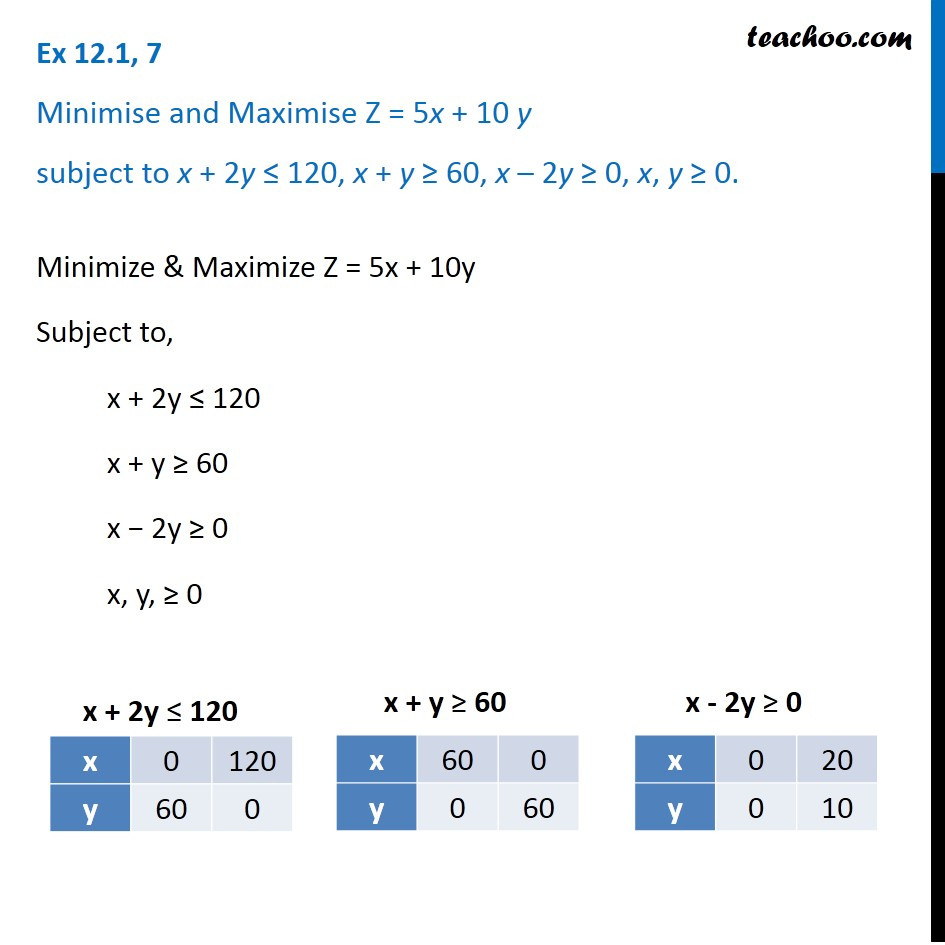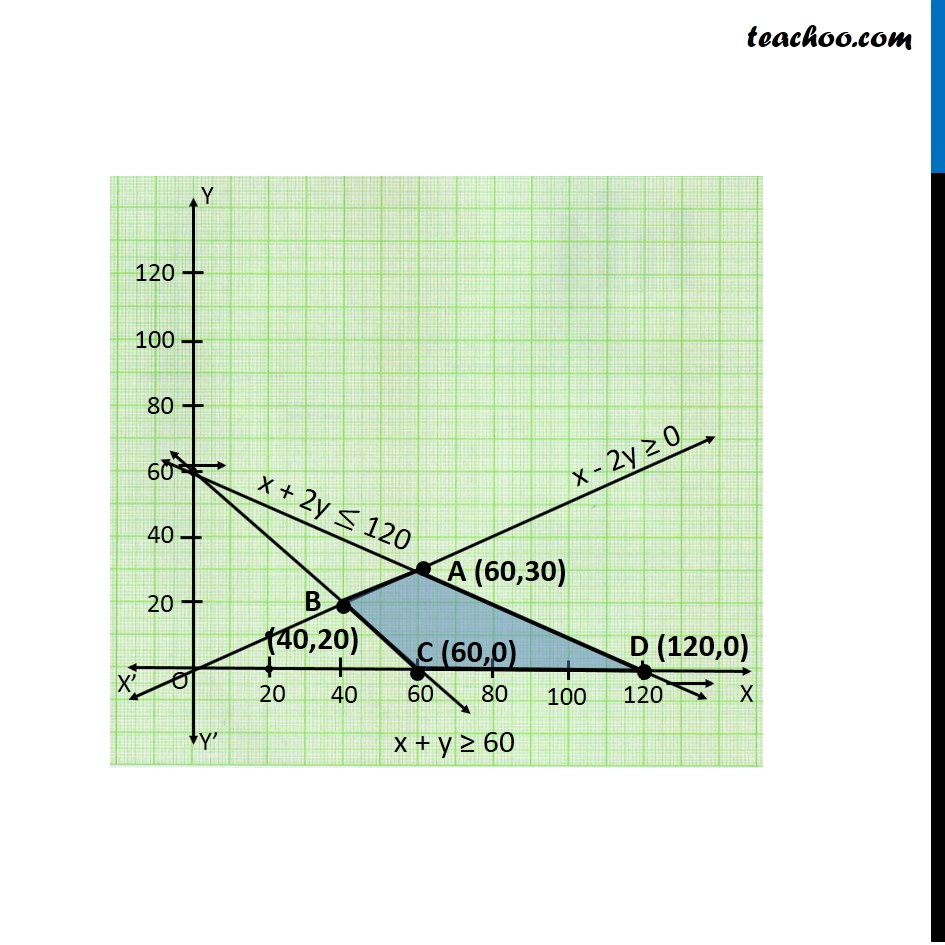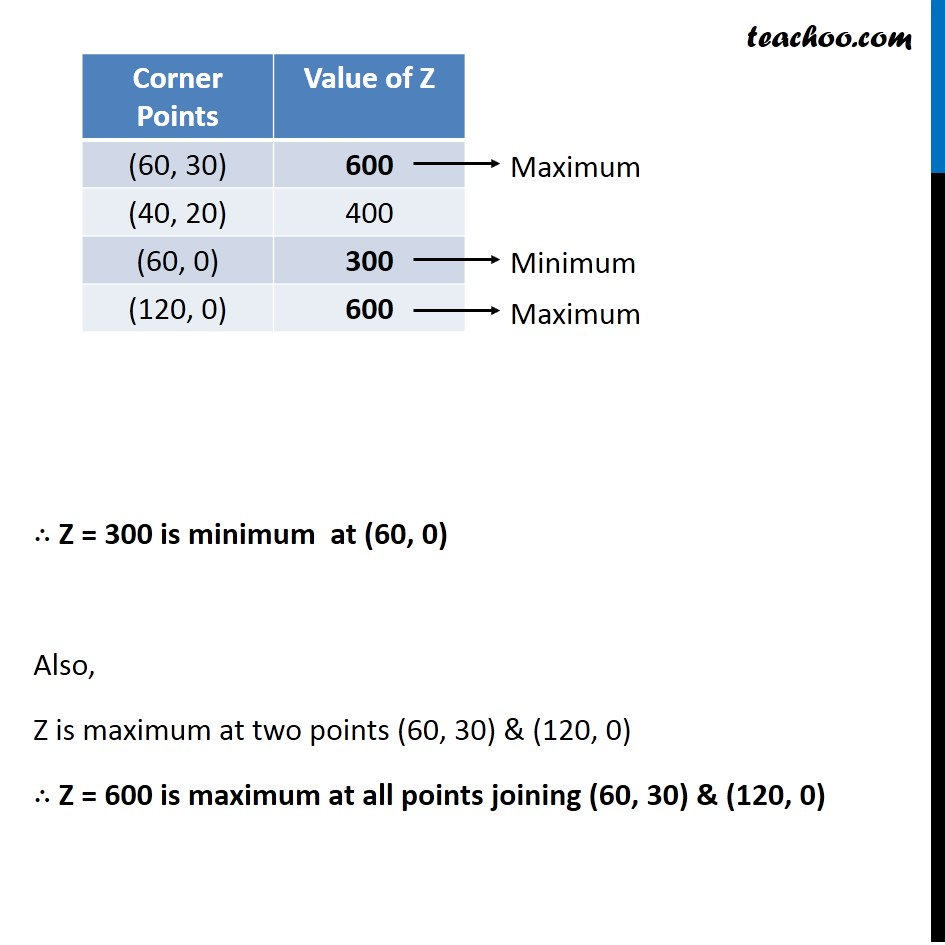Ex 12.1

Chapter 12 Class 12 Linear Programming
Serial order wiseLearn in your speed, with individual attention - Teachoo Maths 1-on-1 Class

### Transcript

Ex 12.1, 7 Minimise and Maximise Z = 5x + 10 y subject to x + 2y ≤ 120, x + y ≥ 60, x – 2y ≥ 0, x, y ≥ 0. Minimize & Maximize Z = 5x + 10y Subject to, x + 2y ≤ 120 x + y ≥ 60 x − 2y ≥ 0 x, y, ≥ 0 ∴ Z = 300 is minimum at (60, 0) Also, Z is maximum at two points (60, 30) & (120, 0) ∴ Z = 600 is maximum at all points joining (60, 30) & (120, 0)